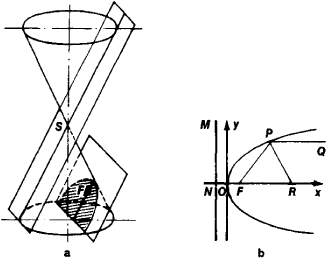# parabola

(redirected from Parabola magazine)
Also found in: Dictionary, Thesaurus.

## parabola

(pərăb`ələ), plane curve consisting of all points equidistant from a given fixed point (focus) and a given fixed line (directrix). It is the conic sectionconic section
or conic
, curve formed by the intersection of a plane and a right circular cone (conical surface). The ordinary conic sections are the circle, the ellipse, the parabola, and the hyperbola.
cut by a plane parallel to one of the elements of the cone. The axis of a parabola is the line through the focus perpendicular to the directrix. The vertex is the point at which the axis intersects the curve. The latus rectum is the chord through the focus perpendicular to the axis. Examples of this curve are the path of a projectile and the shape of the cross section of a parallel beam reflector.

## parabola

(pă-rab -ŏ-lă) A type of conic section with an eccentricity equal to one. See also paraboloid.
Collins Dictionary of Astronomy © Market House Books Ltd, 2006
The following article is from The Great Soviet Encyclopedia (1979). It might be outdated or ideologically biased.

## Parabola

a curve that is the intersection of a circular cone by a plane parallel to a tangent plane to the cone (Figure 1, a); it thus is a conic section. A parabola can also be defined as the locus of points in a plane (Figure 1, b) such that each point is equidistant from a fixed point F of the plane and from a given line MN; F is called the focus and MN the directrix of the parabola. The line passing through the focus perpendicular to the directrix and directed from the directrix toward the focus is the axis of the parabola. The point at which the axis intersects the parabola is the vertex of the parabola.Figure 1

Let us choose the coordinate system xOy, as shown in Figure 1, b. The equation of the parabola then takes the form

y2 = 2px

where p is the length of the segment FN and is called the parameter of the parabola. The parabola is a quadratic curve; it is the graph of the quadratic trinomial y = ax2 + bx + c. It extends to infinity and is symmetric with respect to its axis.

If a light source is placed at the focus of a parabola, the rays reflected by the parabola will form a parallel beam, since the angle formed by the normal PR and the straight line PF connecting any point P of the parabola to the focus is equal to the angle that PR forms with a line parallel to the axis. This property of the parabola is used, for example, in projectors.

## parabola

[pə′rab·ə·lə]
(mathematics)
The plane curve given by an equation of the form y = ax 2+ bx + c.
McGraw-Hill Dictionary of Scientific & Technical Terms, 6E, Copyright © 2003 by The McGraw-Hill Companies, Inc.

## parabola

a conic section formed by the intersection of a cone by a plane parallel to its side. Standard equation: y2 = 4ax, where 2a is the distance between focus and directrix
Collins Discovery Encyclopedia, 1st edition © HarperCollins Publishers 2005
Site: Follow: Share:
Open / Close